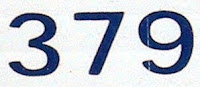## Monday, May 3, 2010

### 379

379 is a prime number.

379 is a divisor of 513 - 1.

379 is the maximum number of regions into which 27 lines divide the plane.

379 is the smallest multidigit prime such that its largest digit is equal to the largest digit of the sum of its digits.

379 is the sum of the seven smallest primes whose last digit is 7: 379 = 7 + 17 + 37 + 47 + 67 + 97 + 107.It was not until page 379 in the first edition of their pivotal Principia Mathematica (a 1910 text deriving logic and mathematics from an axiomatic basis) that Alfred North Whitehead and Bertrand Russell were able to prove the key lemma, which would later allow them to prove that 1 + 1 = 2.